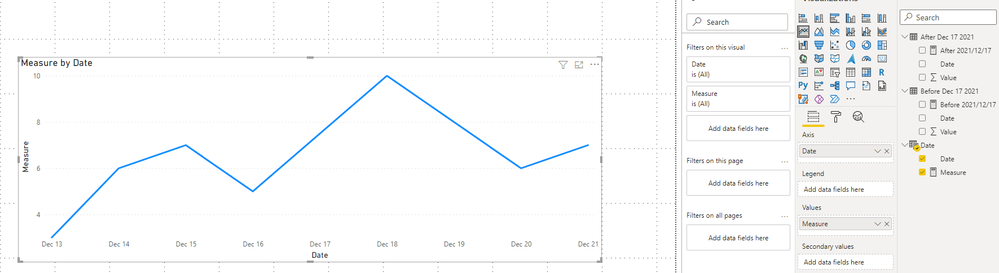cancel
Showing results for
Did you mean:Helper III

## 2 measures in one by date

Hi!

I have 2 measures - one for data before Dec 17 2021 and one for after. We made a change this date so is there away to combine it to one measure so the chart so it's one line before & after the change?

So if I make 2 measures, one called "Pre Dec 17" and one called "Post Dec 17" - how can I show them in one measure called "Combined View" dependent on those dates.

I would think it would be something like

Combined View =

If date is before Dec 17, then use "Pre Dec 17" and if post Dec 17 then use "Post Dec 17"

I hope this makes sense!

Jackie

1 ACCEPTED SOLUTIONCommunity Support

Hi @jnrezk ,

How many data table do you have?

Here are two scenarios:

1. You have two data tables, one for measure before 2021/12/17, another one for measure after 2021/12/17.

2. You have only one data table, it contains all values and you create two measures based on it.

Here I suggest you to create a calendar table with continuous dates by dax. Then relate it with your data table by Date columns. Then create a measure and build a visual by Date column from calendar table.

I think the odds are that you are in situation 1.``````Measure =
IF(MAX('Date'[Date])< DATE(2021,12,17),[Before 2021/12/17],[After 2021/12/17])``````

Result is as below.Best Regards,
Rico Zhou

If this post helps, then please consider Accept it as the solution to help the other members find it more quickly.

7 REPLIES 7Community Support

Hi @jnrezk ,

How many data table do you have?

Here are two scenarios:

1. You have two data tables, one for measure before 2021/12/17, another one for measure after 2021/12/17.

2. You have only one data table, it contains all values and you create two measures based on it.

Here I suggest you to create a calendar table with continuous dates by dax. Then relate it with your data table by Date columns. Then create a measure and build a visual by Date column from calendar table.

I think the odds are that you are in situation 1.``````Measure =
IF(MAX('Date'[Date])< DATE(2021,12,17),[Before 2021/12/17],[After 2021/12/17])``````

Result is as below.Best Regards,
Rico Zhou

If this post helps, then please consider Accept it as the solution to help the other members find it more quickly.Helper III

thanks so much! will give it a trySuper User

@jnrezk , simply add them and try

measure 3 =[measure1] +[Measure2]Helper III

@amitchandak no, i need the logic for the date before and after x date so that the Pre measure doesn't have any data after Dec 17 and the post doesnt pull any data before Dec 17Super User

@jnrezk , comments show option. But if you want to select a date from slicer and what work across all date. Better to use independent date table and measure like

Measure 1=
var _max = maxx(allselected('Date'), 'Date'[Date]) // Or // date(2021,12,17) //Or Today()
return
calculate(sum(Table[value]), filter('Table', 'Table'[Date] > _max))

Measure 2=
var _max = maxx(allselected('Date'), 'Date'[Date]) // Or // date(2021,12,17) //Or Today()
return
calculate(sum(Table[value]), filter('Table', 'Table'[Date] > _max))

or with joined date tbale

Measure 1=
var _max = maxx(allselected('Date'), 'Date'[Date]) // Or // date(2021,12,17) //Or Today()
return
calculate(sum(Table[value]), filter(all('Date'), 'Date'[Date] > _max))

Measure 2=
var _max = maxx(allselected('Date'), 'Date'[Date]) // Or // date(2021,12,17) //Or Today()
return
calculate(sum(Table[value]), filter(all('Date'), 'Date'[Date] > _max))

The second option can not give trendHelper III

I have to be honest you always answer my posts but I never understand hahaSuper User

@jnrezk , Share some sample data and expected output. One More Try